# Quiz 8: Service Facility Location

Huff location model was developed by DL Huff for predicting the benefits that customer can get for a specific store size and its location. This model uses gravity model to find the attractiveness of a facility. Determine the store size and location for AFI using Huff location model as shown below: The maximum size limits for different AFI sites are given below: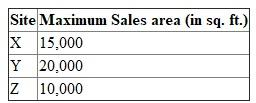Using the above table and minimum travel time data for various sites calculate the attractiveness. Attractiveness to facility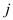for consumer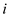is determined using given formula: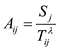…… (1) Here,Here, the value of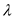is 1. The attractiveness for each site is determined using formula (1) in spreadsheet as shown below: Figure 1: Screenshot of formulas used in excel spreadsheet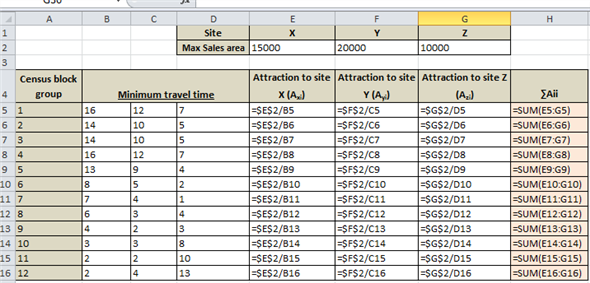Figure 2: Screenshot of results obtained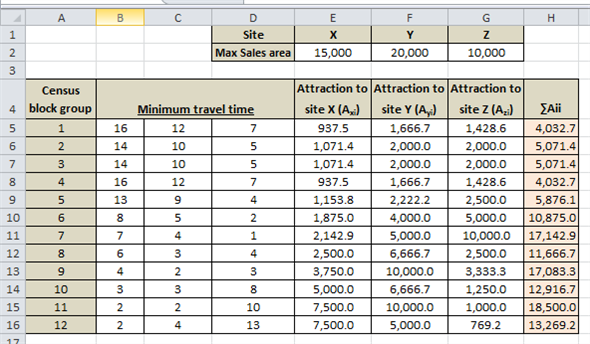Determine the probability of a site using given formula: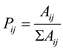…… (2) Here,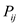is the probability The probability for each site is determined using formula (2) in spreadsheet as shown below: Figure 3: Screenshot of formulas used in excel spreadsheet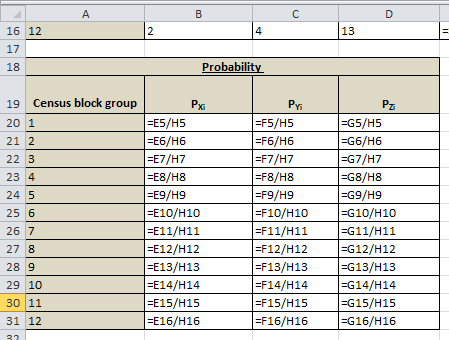Figure 4: Screenshot of results obtained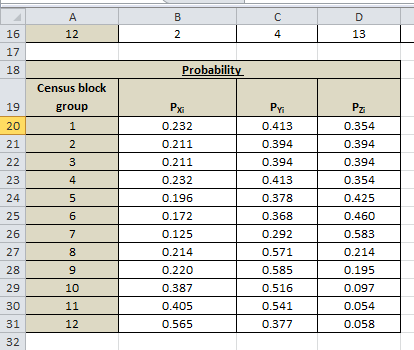Determine the total annual expenditure of a product using given formula: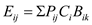…… (3) Here,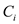= number of customers at area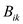= Average annual budget The total annual expenditure for each site is determined using formula (3) in spreadsheet as shown below: Figure 5: Screenshot of formulas used in excel spreadsheet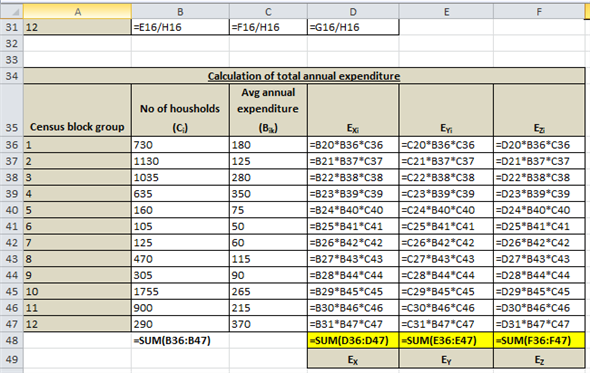Figure 6: Screenshot of results obtained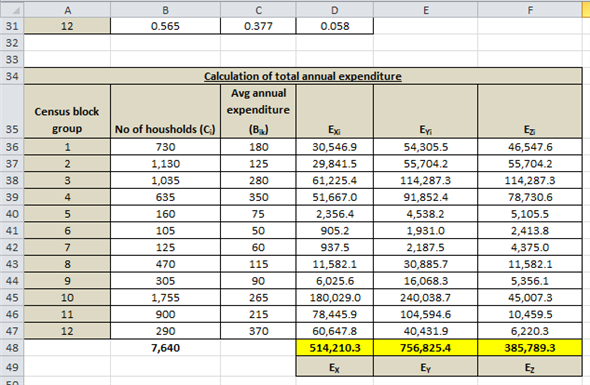Calculate the market share using given formula: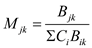…… (4) Here,= number of customers at area= Average annual budget The probability for each site is determined using formula (4) in spreadsheet as shown below: Figure 7: Screenshot of formulas used in excel spreadsheet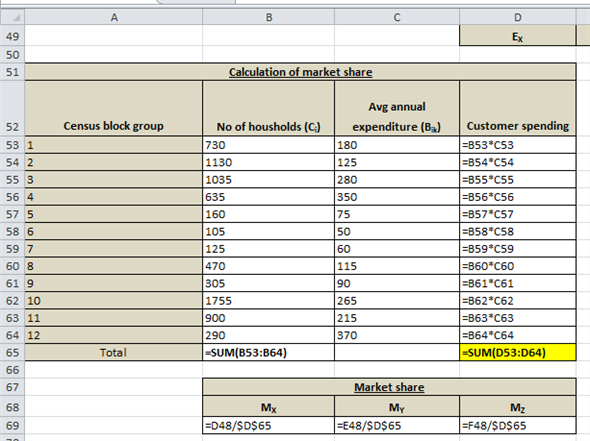Figure 8: Screenshot of results obtained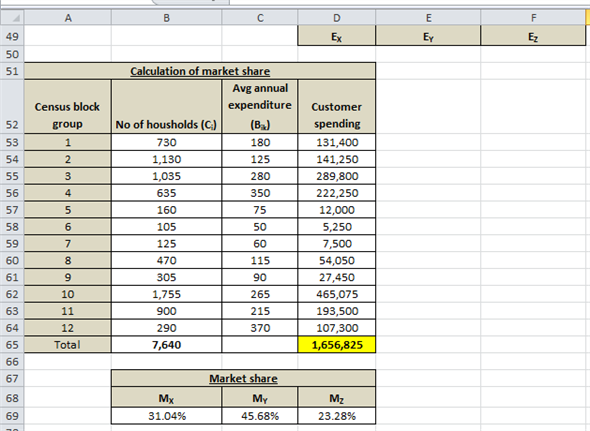Hence, it is seen that market share of Site Y is the highest viz. 45.68%. Therefore, AFI should locate its new store at Site Y. The market share and total annual expenditure of the site are 45.68% and \$756,825 , respectively.
Copying Service Revisited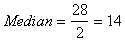No need to relocate because optimal location is line segment (2, 2-3), which includes location A at (2,2).